DraftKings NFC Championship Odds
+300
3 to 1Buccaneers
21.5% implied probability

+450
4.5 to 1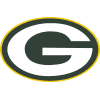Packers
15.6% implied probability

+500
5 to 1Rams
14.3% implied probability

+750
7.5 to 149ers
10.1% implied probability

+850
8.5 to 1Cowboys
9.0% implied probability

+1100
11 to 1Eagles
7.2% implied probability

+2000
20 to 1Cardinals
4.1% implied probability

+2000
20 to 1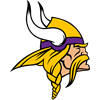Vikings
4.1% implied probability

+2200
22 to 1Saints
3.7% implied probability

+3000
30 to 1Commanders
2.8% implied probability

+6000
60 to 1Panthers
1.4% implied probability

+6000
60 to 1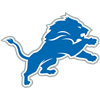Lions
1.4% implied probability

+6000
60 to 1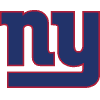Giants
1.4% implied probability

+6500
65 to 1Bears
1.3% implied probability

+7000
70 to 1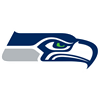Seahawks
1.2% implied probability

+10000
100 to 1Falcons
0.9% implied probability